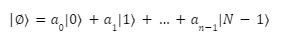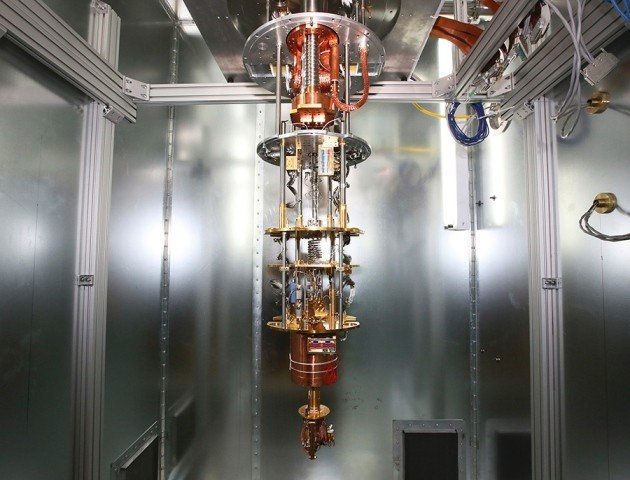Select Page

# What is a Quantum Computer?

by | Jun 27, 2022 | Computing, Quantum Computing

Home » Computing » Quantum Computing » What is a Quantum Computer?

Preface – This post is part of the Quantum Computing series.

## Introduction

So far, we have discussed how Quantum Computing in contrast to Classical Computing. In this module and upcoming modules, we will start to discuss the inner workings of a Quantum Computer. In this module, we will discuss Quantum Processors Units or QPUs and their architectures. In the end, we will demonstrate the inner workings of the D-Wave Quantum Computer. We have much to discuss so, let’s skedaddle!

## What is a Quantum Processing Unit?

A Qubit is the fundamental part of a QPU. All the operations in a QPU are performed on Qubits. Now, we would like to point out at the very beginning that many architectures exist to arrange Qubits on Quantum Processors and all of them have their advantages and drawbacks. As of now, no architecture is proven to be better in terms of efficiency for all kinds of problems. Some promising contending architectures include Superconducting, Photonic, and Trapped ion. Now, we realize these names may sound daunting but just keep a lookout for these in the literature until we eventually discuss each architecture in more detail.

## What is a Quantum Computer?

In the previous sections and modules, we talked about Quantum Computers very abstractly. There were no rigorous proofs or mathematical formulas to actually give you a technical context into the inner workings of Quantum Computers. Here, we will not go all maniac on you with mathematical formulas but we will give you enough to demonstrate our point more clearly. Don’t worry if you don’t understand all of it at once. We advise you to go through this section and do some additional readings we provide at the end of this module.

We will start by defining and representing a Quantum State mathematically. A Quantum State is represented using a vector. The vector represents the probability of the qubit being in that state. A two-qubit system can be represented as shown below.

|0=1 0         |1=0 1

The Quantum States are described using the bra-ket notation. We will discuss it in detail, for now “| ” also known as “ket” is used to specify that 0 and 1 are quantum states.

The vectors representing the quantum states describe the probability of the qubit being in that state. This is a very powerful idea and should be digested as we go further into Quantum Mechanics. Here, the first vector (1 0) describes that the qubit is in the |0 state with probability 1 and |1 state with probability 0. We index the vector starting with 0 and go further along up to the total number of qubits in the system.

Similarly, the second vector (0 1) describes that the qubit is in the |0state with probability 0 and |1state with probability 1.

## Representation of a Quantum State

A pure Quantum state is a superposition of classical states written as,Where, is a complex number representing the probability amplitude of each state in |.

Mathematically, the states |0, . . . , |N-1 form an orthonormal basis of an N-dimensional Hilbert space.

A quantum state | is a vector in this space, usually written as an N-dimensional column vector of its amplitudes:##The picture above shows a D-Wave Quantum Computer. Most of the apparatus you see is used to keep the Quantum Processor situated at the bottom at sub-zero temperature. The Qubits get noisy if the temperature is not maintained which results in Quantum Decoherence. Quantum Decoherence results in errors, noise, and sometimes even loss of information in qubits.

The D-Wave Quantum System is implemented superconducting qubits. There are two models based on which Quantum Computers can be built:

• Quantum Gate Model: In this model, problems are expressed in terms of quantum gates. Companies like Microsoft, IBM, Google, etc. use the Gate Model in their Quantum Computers.
• Quantum Annealing Model: In this model, problems are expressed in terms of optimization problems from which the computer then finds the optimal solution. D-Wave systems use Quantum Annealing to search for solutions in state space.

D-Wave has commercialized its quantum services through its cloud platform Leap. With their latest QPU (Quantum Processing Unit) containing over 5000 qubits, D-Wave is indeed a major player in the Quantum arena.

With this, we conclude this module. In the next module, we will discuss important Quantum Phenomena like Superposition, Entanglement, Interference, and teleportation in detail.

## Author

•A Full Stack Developer with 10+ years of experience in technical content creation.

•